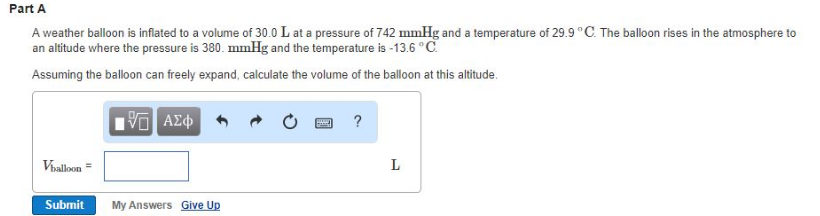# Problem: A weather balloon is inflated to a volume of 30.0 L at a pressure of 742 mmHg and a temperature of 29.9°C. The balloon rises in the atmosphere to an altitude where the pressure is 380. mmHg and the temperature is -13.6°C. Assuming the balloon can freely expand, calculate the volume of the balloon at this altitude.

###### FREE Expert Solution
100% (223 ratings)###### Problem Details

A weather balloon is inflated to a volume of 30.0 L at a pressure of 742 mmHg and a temperature of 29.9°C. The balloon rises in the atmosphere to an altitude where the pressure is 380. mmHg and the temperature is -13.6°C.

Assuming the balloon can freely expand, calculate the volume of the balloon at this altitude.